Example Questions

Example Question #1 : Applying Number Properties

The first 3 prime numbers multiplied together equal:

0

1

30

6

30

Explanation:

The correct answer is 30. A prime number is any number that is only divisible by 1 and itself. 0 and 1 are not prime numbers, so the first 3 prime numbers are 2, 3, and 5.

Example Question #2 : Applying Number Properties

Which of the following numbers is not divisible by 3:

111

412

243

354

412

Explanation:

A common rule about numbers divisible by 3 is that the sum of their digits should be divisible by 3. 4+1+2 = 7 and 7 is not divisible by 3. Therefore, 412 is not divisible by 3.

Example Question #3 : Applying Number Properties

If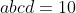and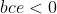, which of the following must be true?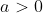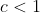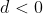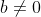Explanation:

Sincebut there are no individual statements about each number on its own, we can’t conclude if a, b, c, or d are positive or negative. Recall that two negative numbers will give a positive product. What we can conclude, though, is that neither a, b, c, or d can equal 0 because then the product would equal 0. This means. A could be greater than 0 or could be less than 0 if either b, c, or d were negative. C could be less than 1, but C could also be a positive number if b or e are negative OR if the product of a, b, and d is positive. Similarly, d could be positive and would support the positive product of 10.

Example Question #4 : Applying Number Properties

Which of the following are rational numbers?

I)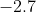II)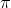III)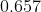II and III

I and III

I, II, and III

I

I and III

Explanation:(pi) is an irrational number. Any square roots or fractions that result in non-terminating, non-repeating decimals fall under the category of irrational numbers.andare both terminating decimals and thus fall under the category of rational numbers.

Example Question #5 : Applying Number Properties

Which of the following are real numbers?

I)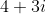II)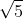III)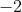I

I, II, and III

II and III

I and II

II and III

Explanation:

Complex numbers (any number in the form of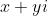) are not real numbers.is irrational, but rational and irrational numbers both fall into the category of real numbers.is an integer, which is a subcategory of real numbers.

Example Question #6 : Applying Number Properties

Which of the following are whole numbers?

I)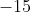II)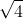III)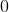II and III

I

I, II, and III

I and II

II and III

Explanation:

Negative rational numbers that don’t have decimals are integers. Whole numbers are a subcategory of integers that only include positive numbers and 0. At first glance, it seems that any square root would fall into irrational numbers, but this particular radical can be simplified to 2, making it a whole number.

Example Question #7 : Applying Number Properties

The correct prime factorization of 210 is?

2, 3, 5, 7

5, 6, 7

3, 7, 10

1, 2, 3, 5, 7

2, 3, 5, 7

Explanation:

Recall that 1 is not a prime number. A prime number is any number greater than 1 that can only be divided by 1 and itself. All the above options are factors of 210, but neither 6 nor 10 are prime numbers (6 can be divided into 2 and 3 while 10 can be divided into 2 and 5).

Example Question #8 : Applying Number Properties

What is the least common multiple of 7, 6, and 10?

105

210

420

42

210

Explanation:

The least common multiple (LCM) refers to the smallest multiple for each number that is shared by all the numbers of a given set, so in this case, it is the smallest multiple of 7, 6, and 10. The best way to approach these problems is to factorize each number and calculate the LCM from the frequency of these numbers. 7 is a prime number, 6 can be factorized into 2 and 3, and 10 can be factorized into 2 and 5. Now, we multiply each factor the greatest number of times it occurs in either number. If the same factor occurs more than once in both numbers, you multiply the factor the greatest number of times it occurs. LCM = 7 * 2 * 3 * 5 = 210. We do not count 2 twice.

Example Question #9 : Applying Number Properties

What is the least common multiple of 30 and 45?

450

270

1350

90

90

Explanation:

The least common multiple (LCM) refers to the smallest multiple for each number that is shared by all the numbers of a given set, so in this case, it is the smallest multiple of 30 and 45. The best way to approach these problems is to factorize each number and calculate the LCM from the frequency of these numbers. 30 can be factorized into 2, 3 and 5. 45 can be factorized into 3, 3, and 5. Now, we multiply each factor the greatest number of times it occurs in either number. If the same factor occurs more than once in both numbers (like it does in 45), you multiply the factor the greatest number of times it occurs. LCM = 2 * 3 * 3 * 5 = 90.

Example Question #10 : Applying Number Properties

What is the greatest common factor of 15 and 75?

3

5

15

30